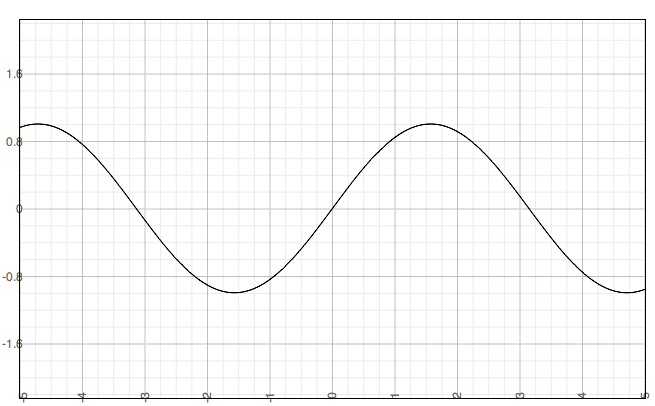SEARCH HOMEMath Central Quandaries & QueriesQuestion from Clara: Please help me to find the domain and range of the following function: F(x)=sin(2x)Hi Clara,

Can you plot the sine function, t = sin(x)?t = sin(s)

Can you answer your question from the graph?

If you need further assistance write back,
HarleyMath Central is supported by the University of Regina and The Pacific Institute for the Mathematical Sciences.